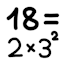#Calculate prime factorization (JavaScript)

## Program

 Number: Factorization:

## Description

This JavaScript program calculates the prime factorization of the given integer. The number must be between 2 and 253.

If the number is very large, the program may hang for a few seconds. This is because the worst-case running time for the number n is O(√n). The algorithm being used is just simple trial division, with a small optimization of skipping even numbers.

The source code is available for viewing.

## Examples

• 7 = 7 (prime)
• 12 = 2 × 2 × 3 = 22 × 3
• 9007199254740881 = 9007199254740881 (largest prime number in range; slow)